2018-05-04

## 优化器框架

TiDB 主要分为两个模块对计划进行优化：

• 逻辑优化，主要依据关系代数的等价交换规则做一些逻辑变换。

• 物理优化，主要通过对查询的数据读取、表连接方式、表连接顺序、排序等技术进行优化。

## 优化器流程

TiDB 一个查询语句的简单流程：一个语句经过 parser 后会得到一个抽象语法树（AST），首先用经过合法性检查后的 AST 生成一个逻辑计划，接着会进行去关联化、谓词下推、聚合下推等规则化优化，然后通过统计数据计算代价选择最优的物理计划，最后执行。流程如下图 1。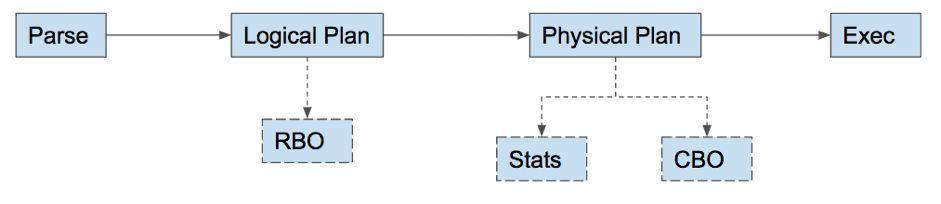### 物理算子简介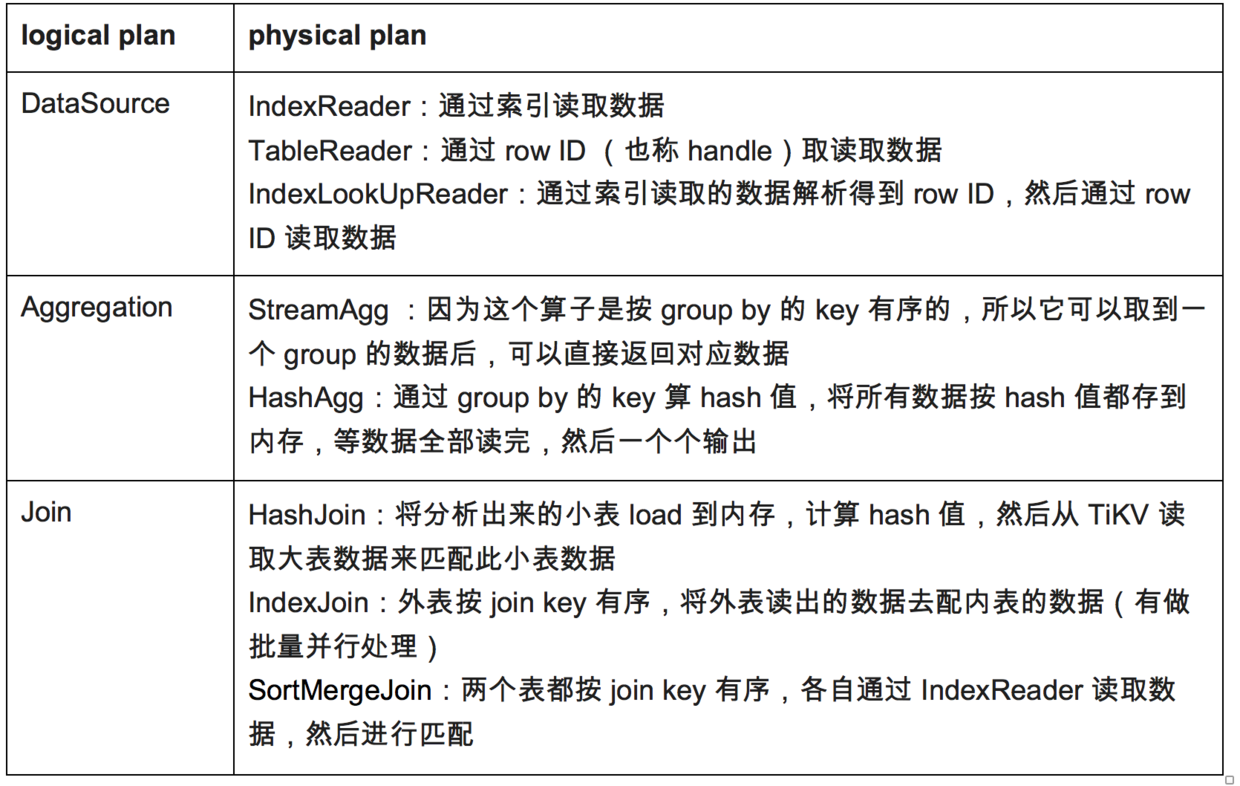## CBO 流程

``````func dagPhysicalOptimize(logic LogicalPlan) (PhysicalPlan,  error) {
logic.preparePossibleProperties()
logic.deriveStats()
if err != nil {
return nil, errors.Trace(err)
}
p  := t.plan()
p.ResolveIndices()
return p, nil
}
``````

• prune prop 对应的函数 preparePossibleProperties。

• 统计信息对应的获取函数 deriveStats。

• 其余章节会介绍函数 convert2PhysicalPlan。

### 例子

``select sum(s.a),count(t.b) from s join t on s.a = t.a and s.c < 100 and t.c > 10 group by s.a``
（其中 a 是索引，b 也是索引）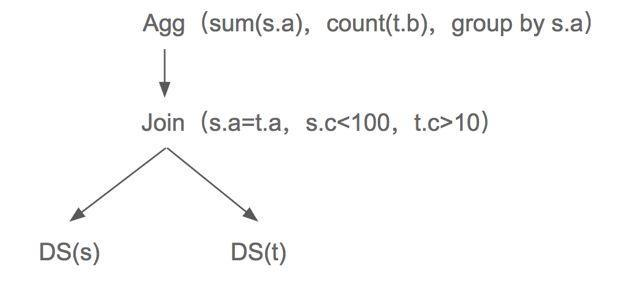TiDB 是用记忆化搜索来处理的。由下往上和由上往下搜索的区别不大，考虑到后者的理解性更好，且按 parent 要求的 prop 传给children，能减少一些可能性（这个后面会具体介绍）。我们选择了由上往下的方式。

``````for _, pp := range p.self.genPhysPlansByReqProp(prop) {
if err != nil {
return nil, errors.Trace(err)
}
}``````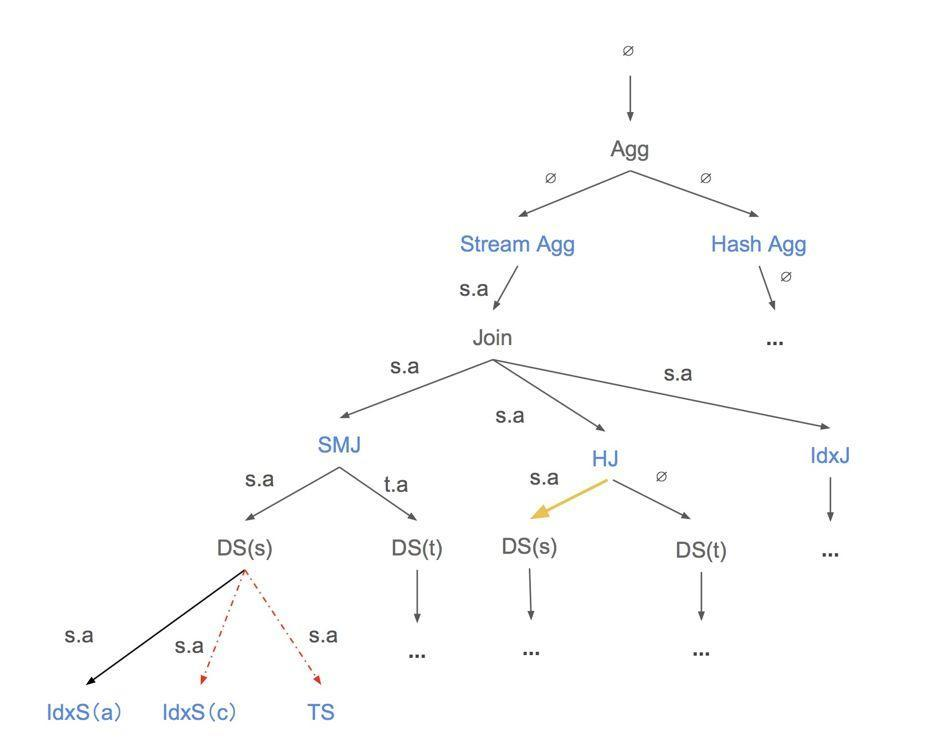（图中黑色字体算子为逻辑算子，蓝色字体为物理算子，黄色箭头为已经计算过代价的算子，会获取已经缓存在哈希表中的结果，红色虚线箭头为不符合 prop 的算子。）

## 代价评估

``````func (p  *baseLogicalPlan)  getBestTask(bestTask task, pp PhysicalPlan) (task, error) {
for i, child := range p.children  {
if err != nil {
return nil, errors.Trace(err)
}
}
}
}``````

### 统计信息

``````// statsInfo stores the  basic information of statistics for the plan's output. It is used for cost  estimation.
type statsInfo struct {
count       float64
cardinality  []float64
}``````

• 方式一，使用真实的统计数据，具体公式如下：
``statsTable.count / histogram.count * hist.NDV``

（statsTable.count 会根据 stats lease 定期更新，histogram.count 只有用户手动 analyze 才更新）

• 方式二，使用一个估计值，由于统计数据在某些情况下还没有收集完成，此时没有统计数据，具体公式如下：
``statsTable.count * distinctFactor``

• DataSource，首先通过前面介绍的两种公式获取 count 和 cardinality，接着用可下推的表达式计算 selectivity 的值，`selectivity = row count after filter / row count before filter`，最后用计算的 selectivity 来调整原来的 count 和 cardinality 的值。

• LogicalJoin（inner join），此算子的 count 获取的公式：

``N(join(s,t)) = N(s) * N(t) / (V(s.key) * V(t.key)) * Min(V(s.key), V(t.key))``
（其中 N 为表的行数，V 为 key 的 cardinality 值）

### expected count

expected count 表示整个 SQL 结束前此算子期望读取的行数。例如 SQL：`select * from s where s.c1 < 5 order by id limit 3` (其中 c1 是索引列，id 是主键列)。我们可以简单认为得到两类可能的计划路径图，如图 4。

• 前者在 PhysicalLimit 时选择 id 有序，那么它的 expected count 为 3。因为有 c1 < 5 的过滤条件，所以在 TableScan 时 expected count 的值为 `min(n(s)，3 / f (σ(c1<5) ))`

• 后者在 TopN 的时候虽然知道它需要读取 3 行，但是它是按 id 列有序，所以它的 expected count 为 Max，在 IndexScan 的时候 expected count 是 `count * f (σ(c1<5)`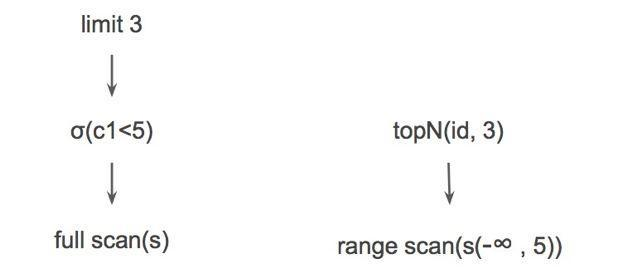``select * from t where c < 1 and b < 1 and a = 1``
(其中 (a,b) 是索引, (b,a,c) 是索引，表 t 有 a、b 和 c 三列)

• doubleread（即IndexLookUpReader ）：`IndexScan( a = 1 and b < 1 ) -> TableScan -> Selection(c < 1)`

• singleread（即IndexReader）：`IndexScan( b < 1 ) -> Selection( a = 1 and c < 1 )`

``Cost(p) = N(p)*FN+M(p)*FM+C(p)*FC``
（其中 N 表示网络开销，M 表示内存开销，C 表示 CPU 开销，F 表示因子）

task 处理的代码主要在文件 `plan/task.go` 中。

## prune properties

``select * from t join s on t.A = s.A and t.B = s.B``

properties 是在 DataSource 这个 logical 算子中获取的，因为此算子中可以得到对应的主键和索引信息。